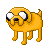###Author Topic: FiM++  (Read 6768 times)

####Rylius

• Regular Member
••• Posts: 60
• Gender:• Silly Filly##### FiM++
« on: 2012-October 05, 08:29:39 »

Somepony implement this for the next MLGJ.####Compony##### Re: FiM++
« Reply #1 on: 2012-October 05, 09:35:38 »
FiM++ Jam - Create an actually usable program in FiM++

####DuckOfDoom##### Re: FiM++
« Reply #2 on: 2012-October 05, 10:21:50 »
Ponify ALL the things!####jigpu##### Re: FiM++
« Reply #3 on: 2012-October 08, 06:53:33 »
FiM++ Jam - Create an actually usable program in FiM++
Does making a program to list all the primes (sans 2) count?JigPu

####Vanni##### Re: FiM++
« Reply #4 on: 2012-October 16, 14:29:10 »

####Oracion

• Regular Member
•• Posts: 12
• Blank flank##### Re: FiM++
« Reply #5 on: 2012-October 16, 21:47:51 »
Well, this was fun. I made a function that calculates the x-th fibonacci number in FiM++.

( public class "A letter about fibonacci numbers" { )
Dear Princess Celestia : A letter about fibonacci numbers

( double fibonacci(double position) { )
I learned the fibonacci number with a number using a number position:

( if (position == 0) { return 1; } )
If position was 0 then:
Then you get 1!
That's what I would do.

( if (position == 1) { return 1; } )
If position was 1 then:
Then you get 1!
That's what I would do.

( double "the previous one" = fibonacci(position - 1); )
Did you know that the previous one is a number?
The previous one is the fibonacci number using position minus 1!

( double "the one before the previous one" = fibonacci(position - 2); )
Did you know that the one before the previous one is a number?
The one before the previous one is the fibonacci number using position minus 2!

( double "the resulting number" = "the previous one" + "the one before the previous one"; )
Did you know that the resulting number is a number?
The resulting number is the previous one added to the one before the previous one!

( return "the resulting number"; )
Then you get the resulting number!

( } )
That's all about the fibonacci number.

( main() { )
Today I learned how to calculate fibonacci numbers recursively:

( double "the position of the desired number"; )
Did you know that the position of the desired number is a number?

( "the position of the desired number" = read(); )
I heard the position of the desired number.

( write(fibonacci("the position of the desired number")); )
I sang the fibonacci number using the position of the desired number.

( } )
That's all about how to calculate fibonacci numbers recursively.

( } )

####Wishdream##### Re: FiM++
« Reply #6 on: 2012-October 17, 11:29:02 »
Wow... that's a lot to type.
Wishdream - Game Developer, Lead of HeoLiX
Game Maker Mentor and Teacher, FM2k2nd Developer, Game Art Designer and Natural Java Programmer
Also, called the Death Developer of the GMC
Part of the IGDA Manila

Hmm... Need something from me? Just PM me okay?
Entered Ludum Dare 2 times.

####Vanni##### Re: FiM++
« Reply #7 on: 2012-October 17, 12:18:27 »
Wow... that's a lot to type.

That's the fun part!More seriously, using FiM++ improve your English in a way, so it's not that bad.

####Rylius

• Regular Member
••• Posts: 60
• Gender:• Silly Filly##### Re: FiM++
« Reply #8 on: 2012-October 17, 23:37:19 »
Here's the most advanced interpreter (apparently): https://github.com/KarolS/fimpp

Just wrote a small Hello Equestria script. Took me waaaay too long to figure out though!